# Rat in a Maze

Amazon, Yahoo, Flipkart, Grofers, MakeMyTrip, Paytm, Visa

Medium

#### Two Solutions Discussed

1. Using recursive backtracking
2. Using iterative backtracking (Using stack)

### Understanding the problem

Given a maze[][] of n * n matrix, a rat has to find a path from source to destination. The left top corner maze is the source, and the right bottom corner maze[n-1][n-1] is the destination. The rat can move in two directions — right and down. In the maze matrix, 0 means the block is a dead end, and 1 means the block can be used in the path from source to destination.

Example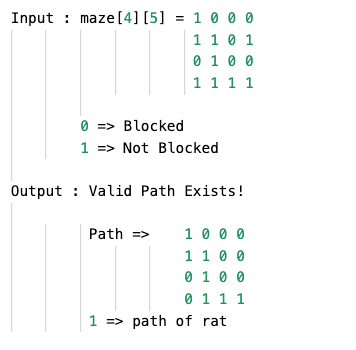## 1. Using Recursive Backtracking

#### Algorithm Idea

Firstly, let us see what backtracking is and how it works and leads us to the solution.

Backtracking: Backtracking is a general algorithm for finding all (or some) solutions to some computational, notably constraint satisfaction problem, that incrementally builds candidates to the solutions and abandons a candidate (“backtracks”) as soon as it determines that the candidate cannot possibly be completed to a correct answer.

So, how does it work on this problem?
If we take a look at the grid, assuming that for every cell where the rat can go, the cell can either lead us to the final cell or not. For every possible move of the rat, if the cell does not lead us to the destination, we can always go back and find other possible solutions.

How do we use this idea?
We will create a solution matrix sol[][] of size n x n to keep track of the rat’s path. Whenever the rat moves to a cell, mark that position in the solution matrix. Now, we will recursively check whether this move leads us to the solution. If this move does not lead us to the solution, we will backtrack or move one step backward and then check for other possible moves.

#### Algorithm Steps

• If the Rat reaches the destination, print the solution matrix.
• Else, check if “isValid(x, y) == true”.
• If true, mark the solution matrix [x][y] = 1.
if(solveMaze(x + 1, y) == true)
return true.
if(solveMaze(x, y + 1) == true)
return true.
Else, mark solution matrix [x][y] = 0 and return false (Backtrack).
• Else, return false.

#### Algorithm Pseudo Code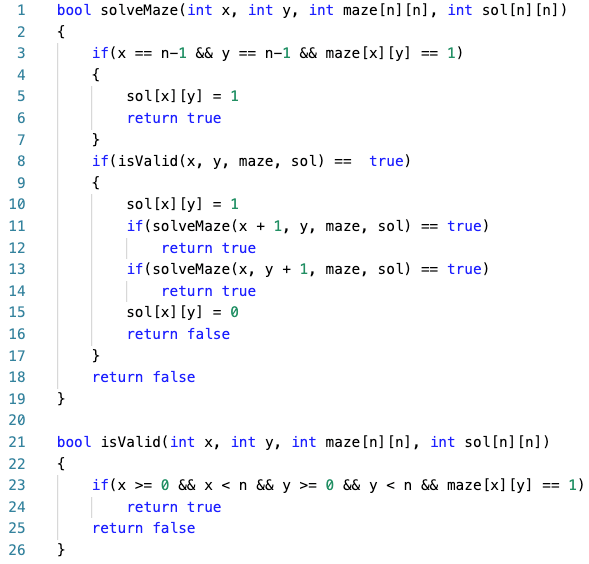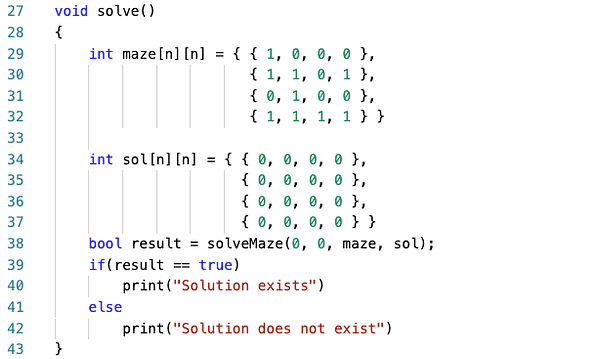#### Algorithm Analysis

The number of moves possible for the rat is two. The algorithm can go down and go right and then try out every possibility to fill the lower square. Time Complexity for the backtracking solution = O(2^(n²)) because we need to consider two different paths at every position.

Space complexity is proportional to the matrix's size, which is required to keep track of the solution matrix, i.e., O(n²).

## 2. Using iterative backtracking (Using stack)

We will use a stack to keep track of the recursive calls in the above algorithm. Using a node, we will maintain the position and direction of the move. The main crux behind this algorithm is to push a particular node, and for every node on the top of the Stack, we will try out every possible move until we find a solution or return false. Also, we will check if we have already visited a particular cell to reduce the time complexity.

#### Algorithm Steps

1. Create a node having three variables for position and direction.
node{ int x, y, dir = 0; }.
2. With the help of a constructor, pass the position and direction of the current cell.
3. Now, declare a stack and push the source node.
4. Perform this while the Stack is not empty:
i. Using new variables, store the top of the Stack (x, y, and dir), then pop the Stack and increment the direction. Push the node again to the Stack.
ii. Now, if the current cell is the destination, return true.
iii. Else, check right and down directions.
iv. If no path leads to the last cell, the backtrack by making the current cell unvisited.
5. If we cannot reach the final solution, return false.

#### Algorithm Pseudo Code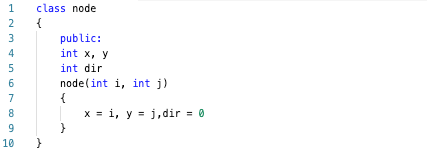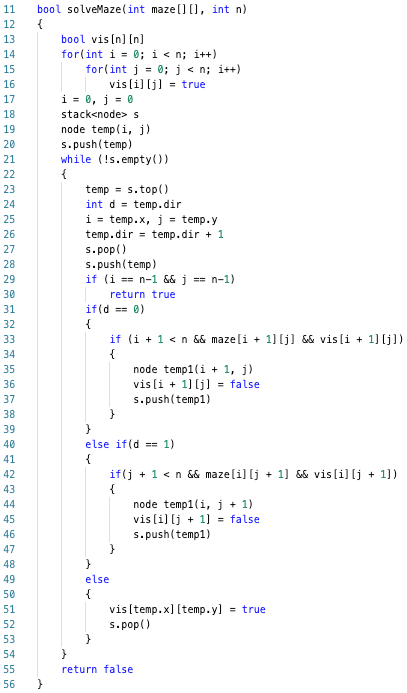#### Algorithm Analysis

☆ 16-Week Live DSA Course
☆ 10-Week Live DSA Course